# You think you have problems well we have

• Slides: 76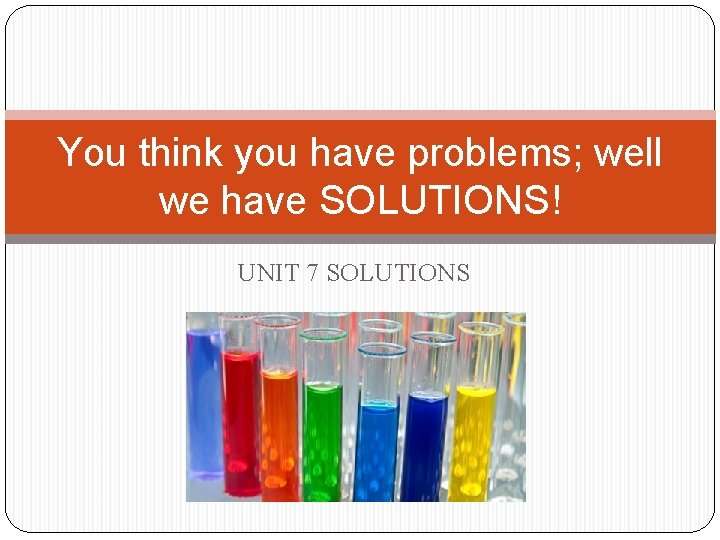You think you have problems; well we have SOLUTIONS! UNIT 7 SOLUTIONSWhy does a raw egg swell or shrink when placed in different solutions?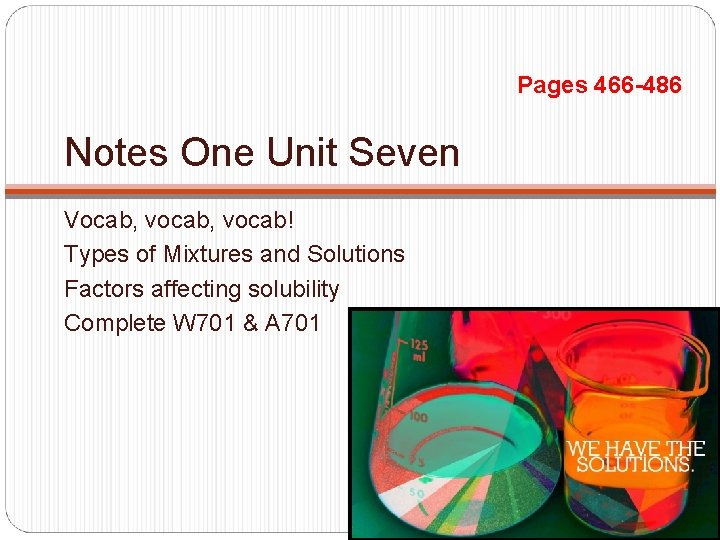Pages 466 -486 Notes One Unit Seven Vocab, vocab! Types of Mixtures and Solutions Factors affecting solubility Complete W 701 & A 701Important Vocabulary Solutions are simply a homogenous mixture Homogenous: same throughout Ex: Water + Sugar, Air Heterogeneous: different throughout Ex: Oil+ Water Solutions= Solute + Solvent Solute Things that get dissolved Solvent Things into which solute is dissolved Determines the state of solution Largest componentCommon Mixtures SOLUTE SOLVENT Type EXAMPLE liquid mayonnaise liquid emulsion gas liquid foam whipped cream solid dust in air gas aerosol liquid hair spray gas aerosol solid ruby glass solid liquid pearl solid emulsion gas solid foam StyrofoamSolution Types SOLUTE SOLVENT PHASE gas gas liquid liquid solid solid EXAMPLE air soda pop antifreeze filling seawater brass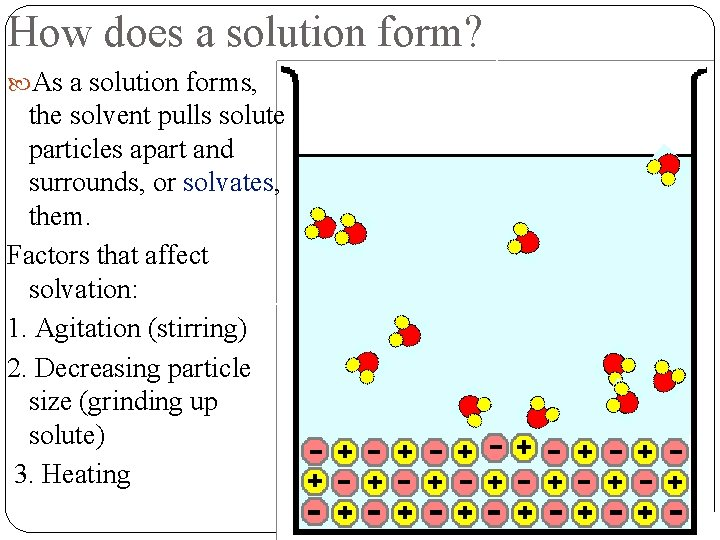How does a solution form? As a solution forms, the solvent pulls solute particles apart and surrounds, or solvates, them. Factors that affect solvation: 1. Agitation (stirring) 2. Decreasing particle size (grinding up solute) 3. HeatingKey Difference Solvation Solubility Process of dissolving The amount of solute Rate of dissolving- that can be dissolved in a given amount of solvent Amount that can be dissolved-HOW MUCH HOW FAST RATE of dissolving is not the same as the AMOUNT that can be dissolved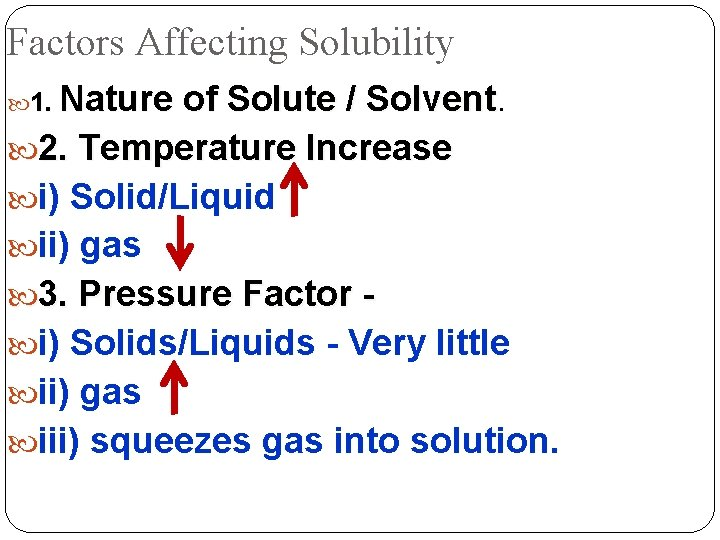Factors Affecting Solubility 1. Nature of Solute / Solvent 2. Temperature Increase i) Solid/Liquid ii) gas 3. Pressure Factor i) Solids/Liquids - Very little ii) gas iii) squeezes gas into solution.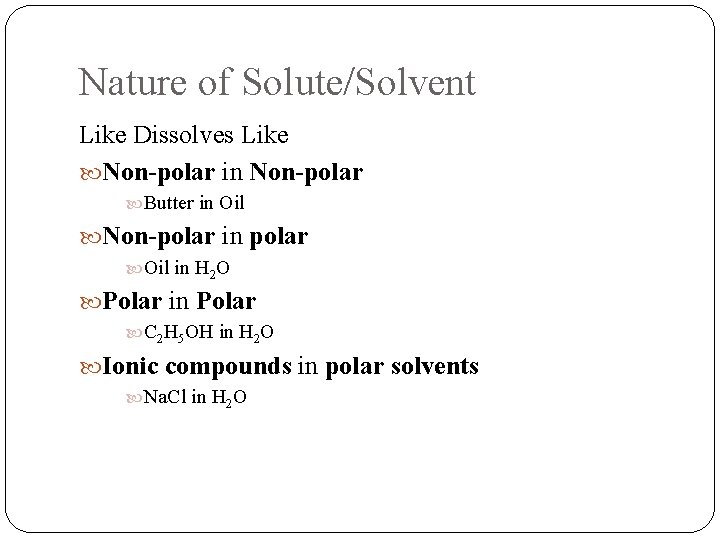Nature of Solute/Solvent Like Dissolves Like Non-polar in Non-polar Butter in Oil Non-polar in polar Oil in H 2 O Polar in Polar C 2 H 5 OH in H 2 O Ionic compounds in polar solvents Na. Cl in H 2 OAt Higher Temperatures…. Gases become less soluble in water Ex: Boiling water. The first bubbles that appear when tap water is heated are bubbles of air released as the increasing temperature reduces the solubility of air in water. Most ionic solids become more soluble in water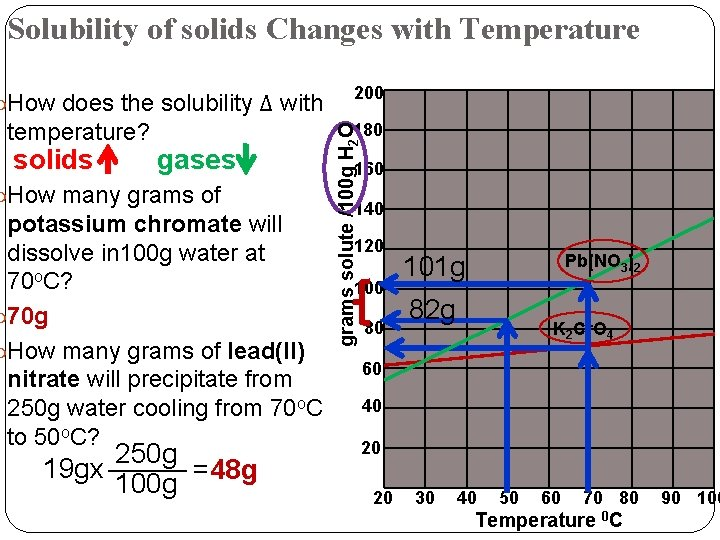Solubility of solids Changes with Temperature How does the solubility Δ with solids 180 gases How many grams of potassium chromate will dissolve in 100 g water at 70 o. C? 70 g How many grams of lead(II) nitrate will precipitate from 250 g water cooling from 70 o. C to 50 o. C? 250 g _____ 19 gx =48 g 100 g grams solute /100 g H 2 O temperature? 200 160 140 120 100 80 101 g Pb(NO 3)2 82 g K 2 Cr. O 4 60 40 20 20 30 40 50 60 70 80 Temperature 0 C 90 100Solubility of Gases Changes with Temperature 5. 0 4. 5 milligrams solute /100 g H 2 O a) Why are fish stressed, if the temperature of the water increases? How much does the solubility of oxygen change, for a 20 o. C to 60 o. C change? 1. 00 -0. 60=0. 40 mg 4. 0 3. 5 3. 0 2. 5 2. 0 1. 5 1. 00 mg 0. 60 mg 1. 0 O 2 N 2 0. 5 0 10 20 30 40 50 60 Temperature 0 C 70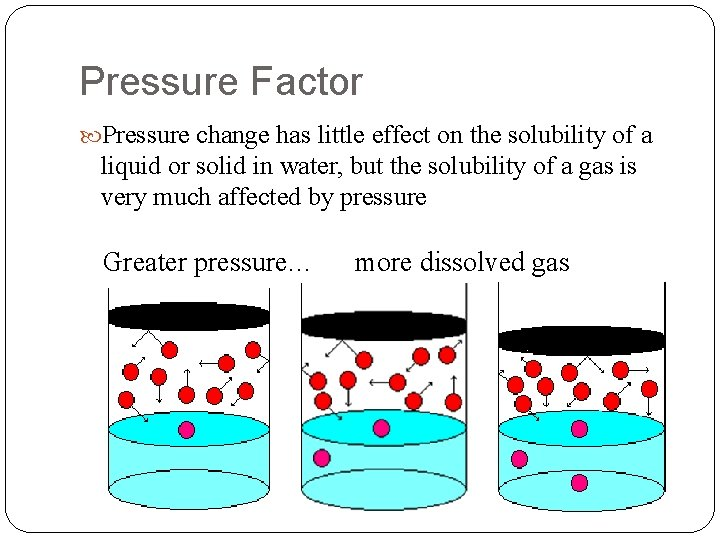Pressure Factor Pressure change has little effect on the solubility of a liquid or solid in water, but the solubility of a gas is very much affected by pressure Greater pressure… more dissolved gasSummary Solutions are a homogenous mixture Solutions are made up of solutes and solvents Solubility refers to the how well solutes dissolve in solvents Solvation refers to the process of dissolving Agitation, heating, and decreasing particle size affect the rate of solvation Factors that affect solubility: Nature of solvents Temperature Pressure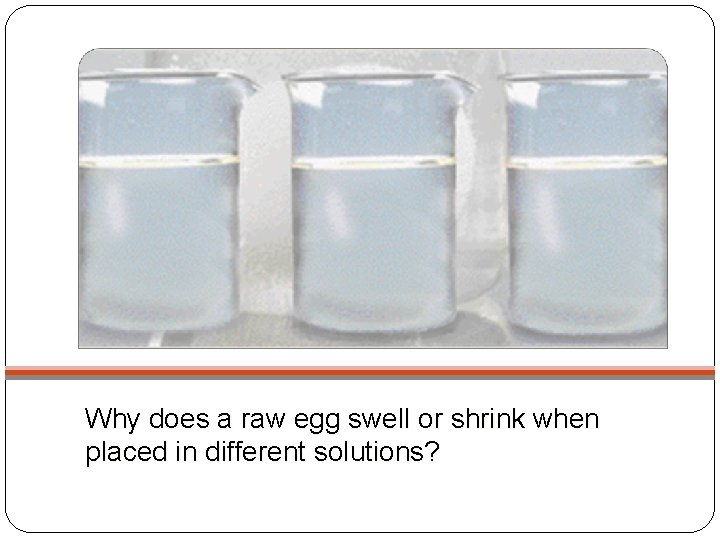Why does a raw egg swell or shrink when placed in different solutions?An egg soaking in vinegar with part of it’s shell dissolved. A dehydrated naked egg side-by-side with a brilliant red naked egg soaked in red food coloring. A naked egg that was soaked in corn syrup for a few days. Much of the water inside the egg has moved out through the membrane.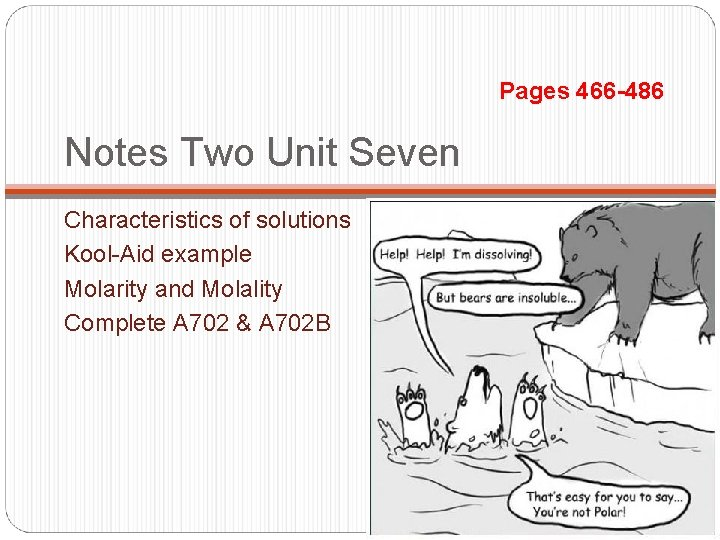Pages 466 -486 Notes Two Unit Seven Characteristics of solutions Kool-Aid example Molarity and Molality Complete A 702 & A 702 B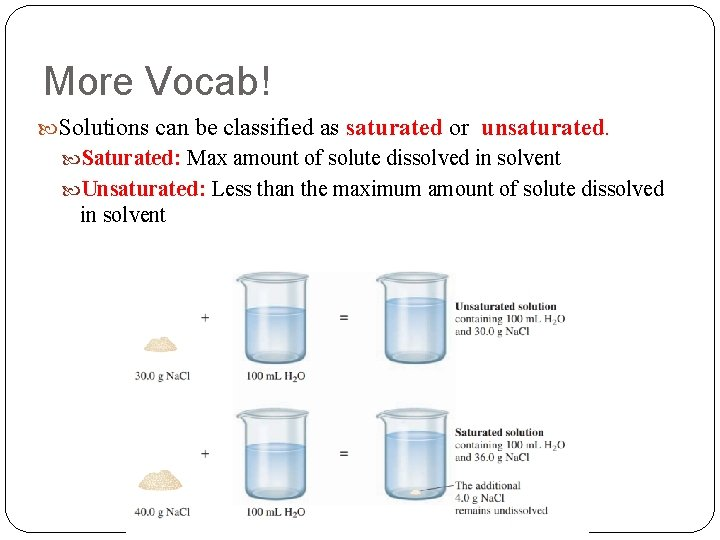More Vocab! Solutions can be classified as saturated or unsaturated. Saturated: Max amount of solute dissolved in solvent Unsaturated: Less than the maximum amount of solute dissolved in solvent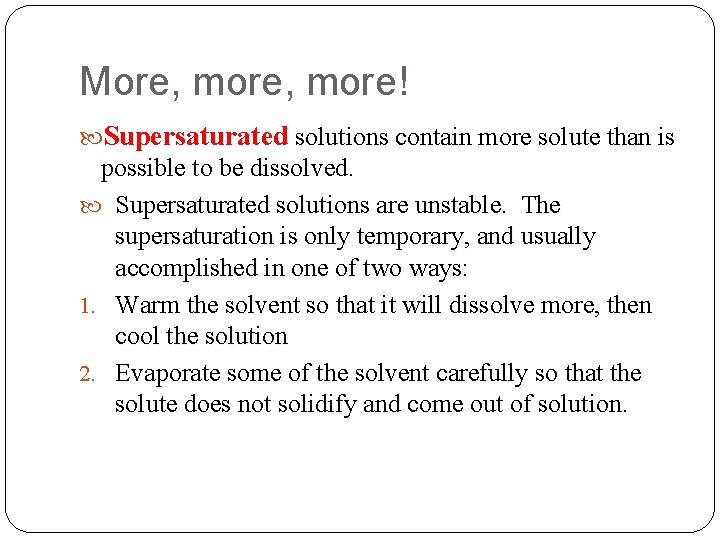More, more! Supersaturated solutions contain more solute than is possible to be dissolved. Supersaturated solutions are unstable. The supersaturation is only temporary, and usually accomplished in one of two ways: 1. Warm the solvent so that it will dissolve more, then cool the solution 2. Evaporate some of the solvent carefully so that the solute does not solidify and come out of solution.Characteristics of SolutionsSolutions can also be described as Concentrated This means that there is a lot of solute dissolved per amount of solvent. Dilute This means that there is relatively little solute dissolved per amount of solvent.Making Kool-Aid When you make Kool-Aid, what is the solute? What is the solvent? What is the solution?Making Kool-Aid If you make a concentrated solution of Kool-Aid. solute This solution has too much______. If you make a dilute solution of Kool-Aid. solvent This solution has too much ______. How can we use the concentrated solution of Kool-Aid to make a more dilute solution of Kool. Aid?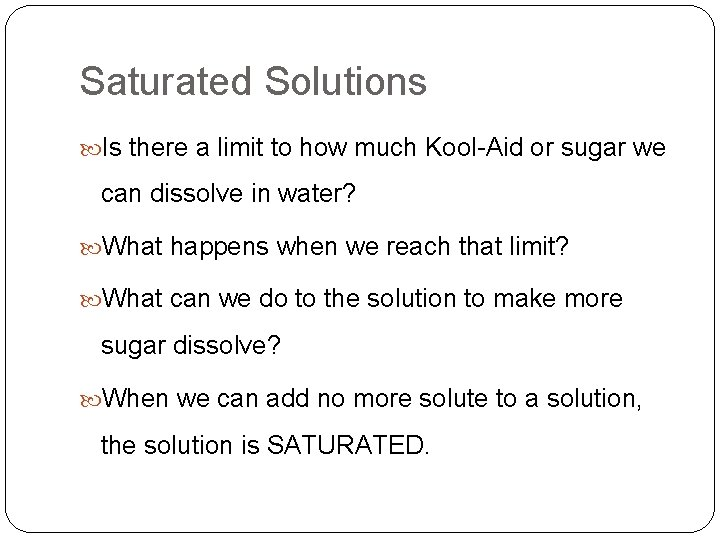Saturated Solutions Is there a limit to how much Kool-Aid or sugar we can dissolve in water? What happens when we reach that limit? What can we do to the solution to make more sugar dissolve? When we can add no more solute to a solution, the solution is SATURATED.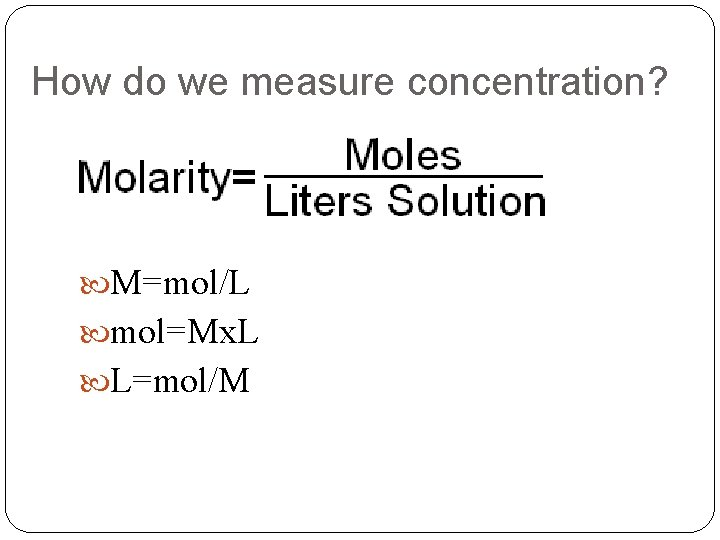How do we measure concentration? M=mol/L mol=Mx. L L=mol/M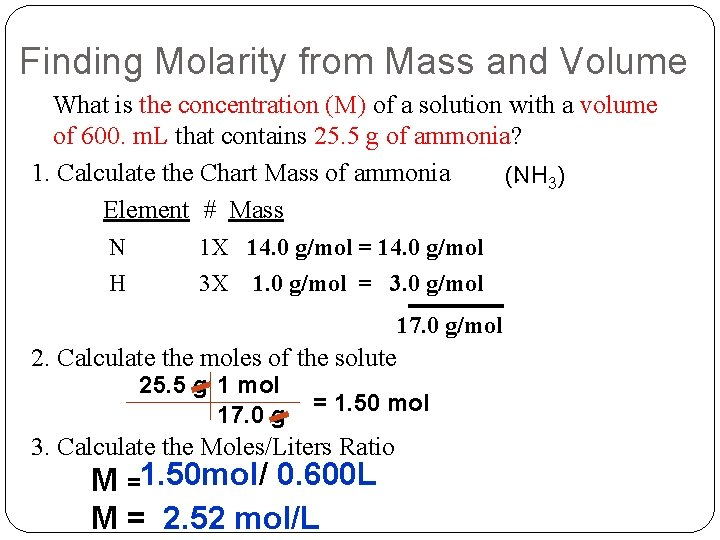Finding Molarity from Mass and Volume What is the concentration (M) of a solution with a volume of 600. m. L that contains 25. 5 g of ammonia? 1. Calculate the Chart Mass of ammonia : 3) (NH Element # Mass N H 1 X 14. 0 g/mol = 14. 0 g/mol 3 X 1. 0 g/mol = 3. 0 g/mol 17. 0 g/mol 2. Calculate the moles of the solute 25. 5 g 1 mol 17. 0 g = 1. 50 mol 3. Calculate the Moles/Liters Ratio M =1. 50 mol/ 0. 600 L M = 2. 52 mol/L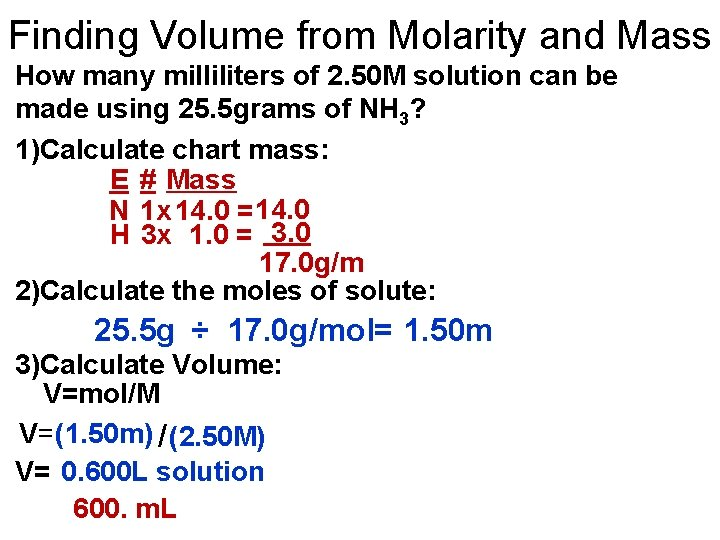Finding Volume from Molarity and Mass How many milliliters of 2. 50 M solution can be made using 25. 5 grams of NH 3? 1)Calculate chart mass: E # Mass N 1 x 14. 0 = 14. 0 H 3 x 1. 0 = 3. 0 17. 0 g/m 2)Calculate the moles of solute: 25. 5 g ÷ 17. 0 g/mol= 1. 50 m 3)Calculate Volume: V=mol/M V=(1. 50 m) / (2. 50 M) V= 0. 600 L solution 600. m. L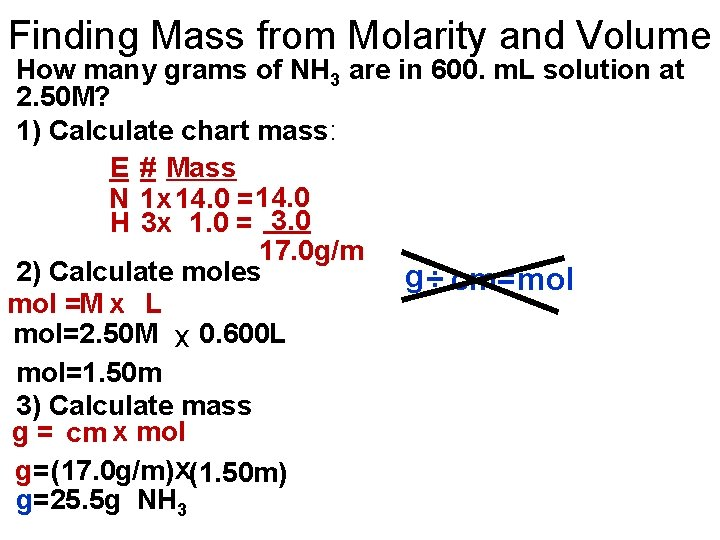Finding Mass from Molarity and Volume How many grams of NH 3 are in 600. m. L solution at 2. 50 M? 1) Calculate chart mass: E # Mass N 1 x 14. 0 = 14. 0 H 3 x 1. 0 = 3. 0 17. 0 g/m 2) Calculate moles g ÷ cm=mol =M x L mol=2. 50 M x 0. 600 L mol=1. 50 m 3) Calculate mass g = cm x mol g=(17. 0 g/m)x(1. 50 m) g=25. 5 g NH 3We also measure concentration with Molality (m) • Is defined as : MOLES OF SOLUTE Kg of SOLVENTMolality, what? Why in the world would we have a separate way of expressing concentration, the equation for which looks so similar to the molarity equation? The answer lies in the denominator of that equation. Molality does not change with temperature of the solution. While volume of a solution can change with temperature due to expansion or contraction, the mass of the solvent will not change with temperature.Finding Molality from Mass What is the molality (m) of a solution that has 18. 7 grams of magnesium sulfate in 550 grams of water? 1. Calculate the Chart Mass of magnesium sulfate Element # Mass Mg S O 1 X 24. 3 g/mol = 24. 3 g/mol 1 X 32. 0 g/mol = 32. 0 g/mol 4 X 16. 0 g/mol = 64. 0 g/mol 120. 3 g/mol 2. Calculate the moles of the solute 18. 7 g 1 mol =. 921 mol 120. 3 g 4. Calculate Molality (m) m=. 921 mol/. 55 kg= 1. 67 mol/kg (Mg. SO 4) 3. Convert grams to kilograms 550 g. 001 kg 1 g =. 55 kg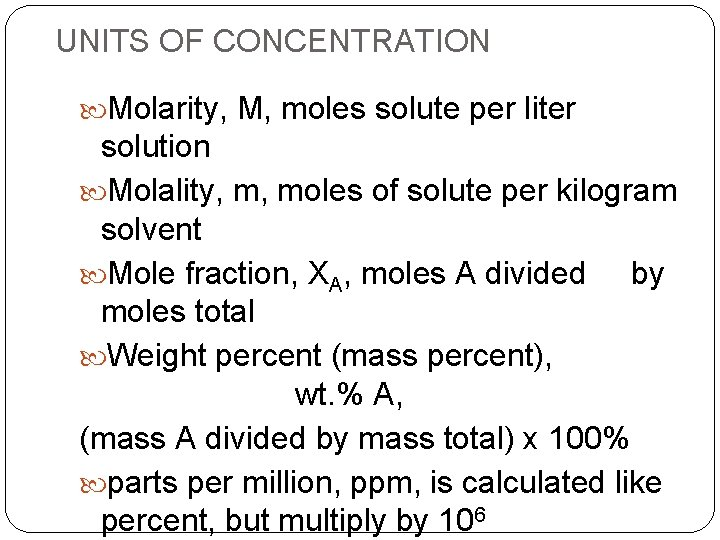UNITS OF CONCENTRATION Molarity, M, moles solute per liter solution Molality, m, moles of solute per kilogram solvent Mole fraction, XA, moles A divided by moles total Weight percent (mass percent), wt. % A, (mass A divided by mass total) x 100% parts per million, ppm, is calculated like percent, but multiply by 106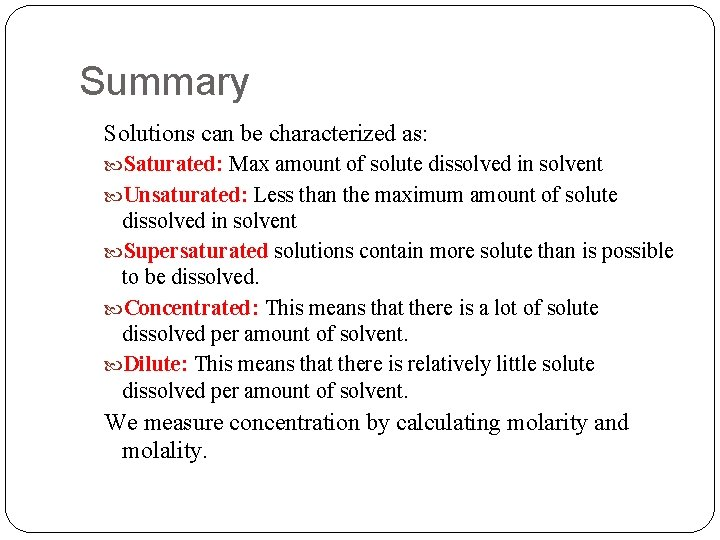Summary Solutions can be characterized as: Saturated: Max amount of solute dissolved in solvent Unsaturated: Less than the maximum amount of solute dissolved in solvent Supersaturated solutions contain more solute than is possible to be dissolved. Concentrated: This means that there is a lot of solute dissolved per amount of solvent. Dilute: This means that there is relatively little solute dissolved per amount of solvent. We measure concentration by calculating molarity and molality.Notes Three Unit Seven Dilutions Complete L 701 Compete D 701 Flint articlesDilutions Relatively little solute dissolved per amount of solvent. We care about dilutions because sometimes it is necessary to prepare a solution from an existing solution Solution of Na. Cl vs solution of HCl(aq) Use this formula to determine how to dilute a stock solution to the desired concentration: M 1 V 1 = M 2 V 2Dilutions Lab Purpose: To perform a serial dilution using a solution of the ionic compound potassium permanganate (KMn. O 4).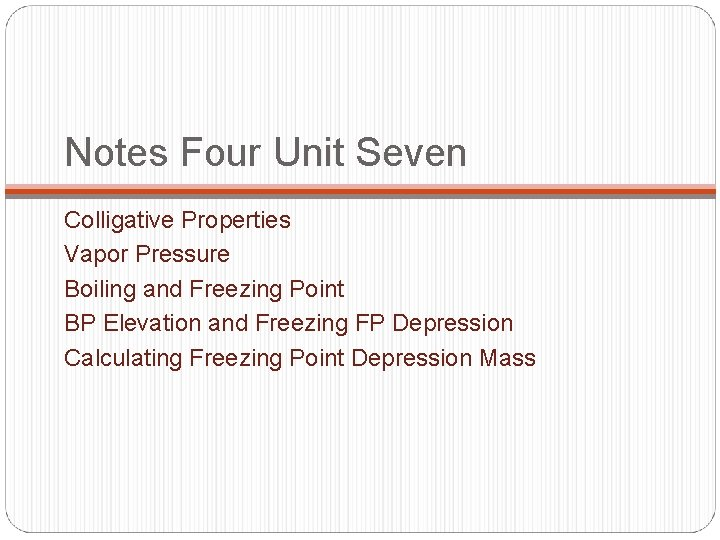Notes Four Unit Seven Colligative Properties Vapor Pressure Boiling and Freezing Point BP Elevation and Freezing FP Depression Calculating Freezing Point Depression MassHow do you get from this……to this?Add an ionic compound!Colligative Properties How solutes affect the properties of solutionsColligative Properties On adding a solute to a solvent, the properties of the solvent are modified. Vapor pressure Boiling point Freezing point decreases (lowering) increases (elevates) decreases (depression) These changes are called COLLIGATIVE PROPERTIES. They depend only on the NUMBER of solute particles relative to solvent particles, not on the KIND of solute particles.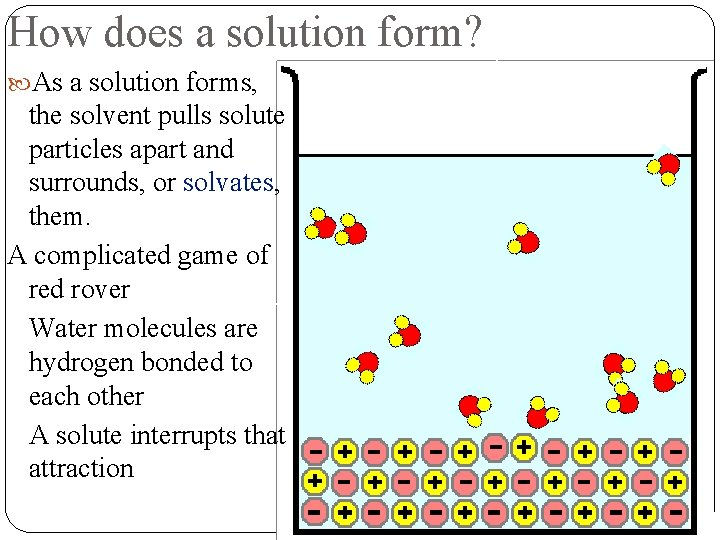How does a solution form? As a solution forms, the solvent pulls solute particles apart and surrounds, or solvates, them. A complicated game of red rover Water molecules are hydrogen bonded to each other A solute interrupts that attraction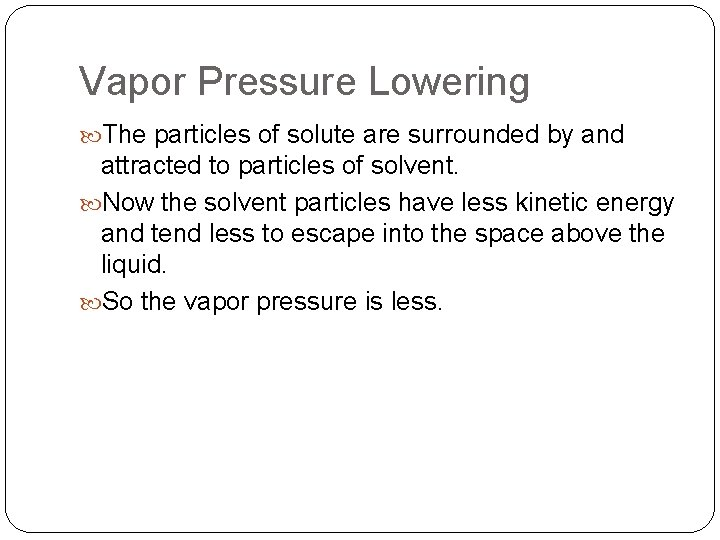Vapor Pressure Lowering The particles of solute are surrounded by and attracted to particles of solvent. Now the solvent particles have less kinetic energy and tend less to escape into the space above the liquid. So the vapor pressure is less.Why does vapor pressure of the solvent decrease?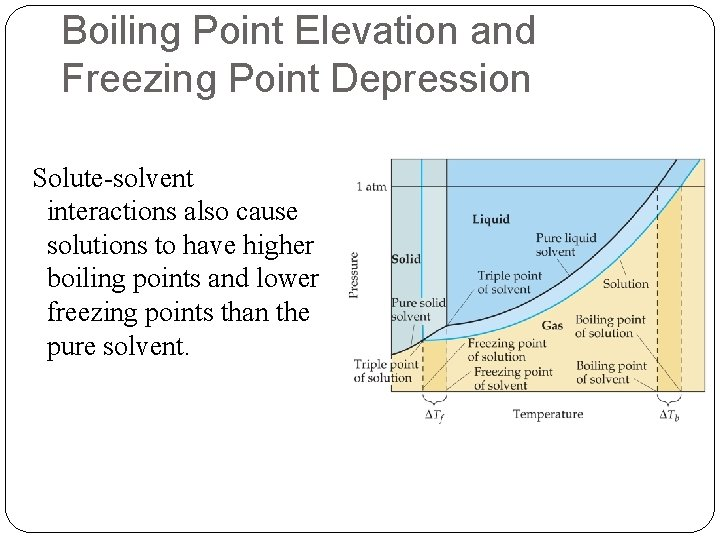Boiling Point Elevation and Freezing Point Depression Solute-solvent interactions also cause solutions to have higher boiling points and lower freezing points than the pure solvent.Effect on Freezing Point The addition of a solute to a solvent causes the freezing point of the solution to decrease.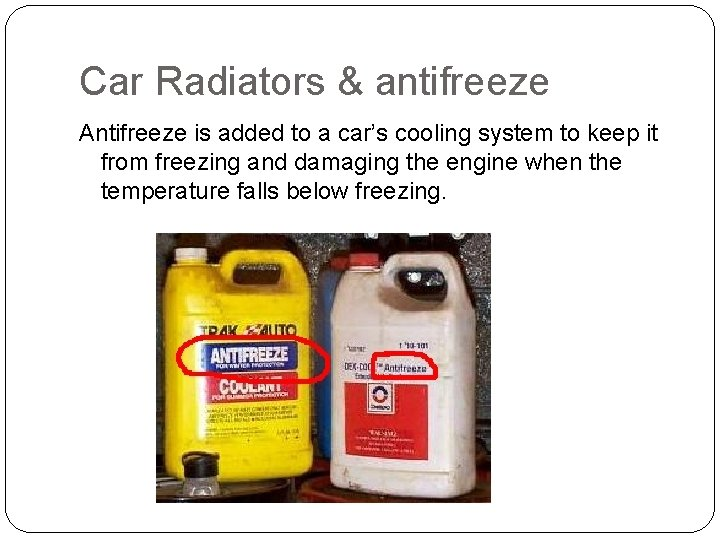Car Radiators & antifreeze Antifreeze is added to a car’s cooling system to keep it from freezing and damaging the engine when the temperature falls below freezing.Definition of Boiling Point The temperature at which the vapor pressure of a liquid becomes equal to the pressure above the liquid.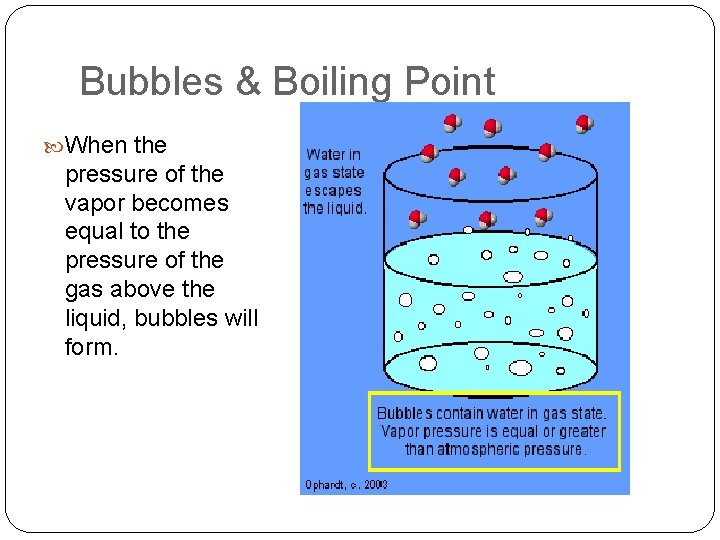Bubbles & Boiling Point When the pressure of the vapor becomes equal to the pressure of the gas above the liquid, bubbles will form.Boiling Point Elevation The change in boiling point is proportional to the molality of the solution: Tb = Kb m where Kb is the molal boiling point elevation constant, a property of the solvent. Tb is added to the normal boiling point of the solvent.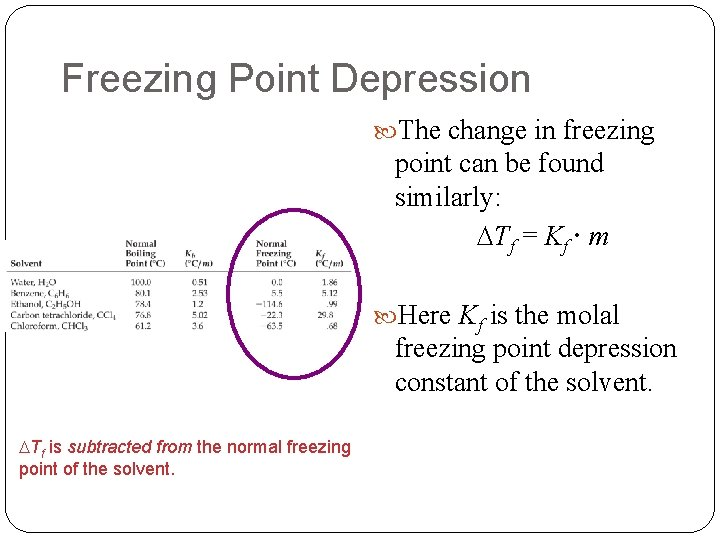Freezing Point Depression The change in freezing point can be found similarly: Tf = Kf m Here Kf is the molal freezing point depression constant of the solvent. Tf is subtracted from the normal freezing point of the solvent.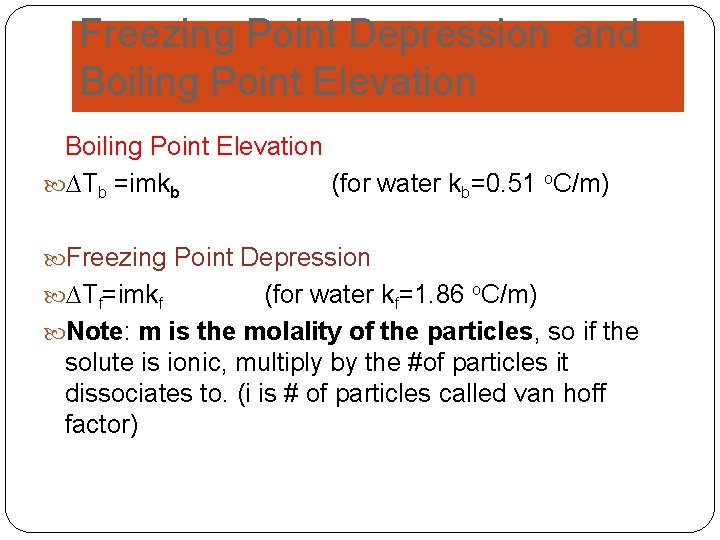Freezing Point Depression and Boiling Point Elevation ∆Tb =imkb (for water kb=0. 51 o. C/m) Freezing Point Depression ∆Tf=imkf (for water kf=1. 86 o. C/m) Note: m is the molality of the particles, so if the solute is ionic, multiply by the #of particles it dissociates to. (i is # of particles called van hoff factor)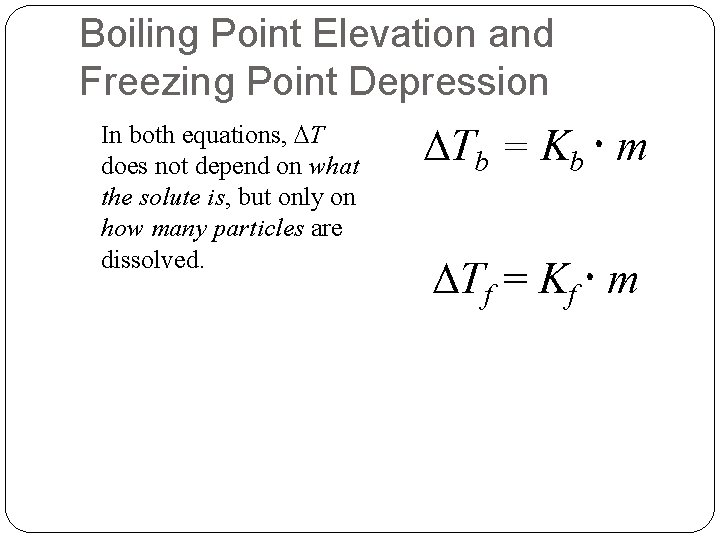Boiling Point Elevation and Freezing Point Depression In both equations, T does not depend on what the solute is, but only on how many particles are dissolved. Tb = Kb m Tf = Kf m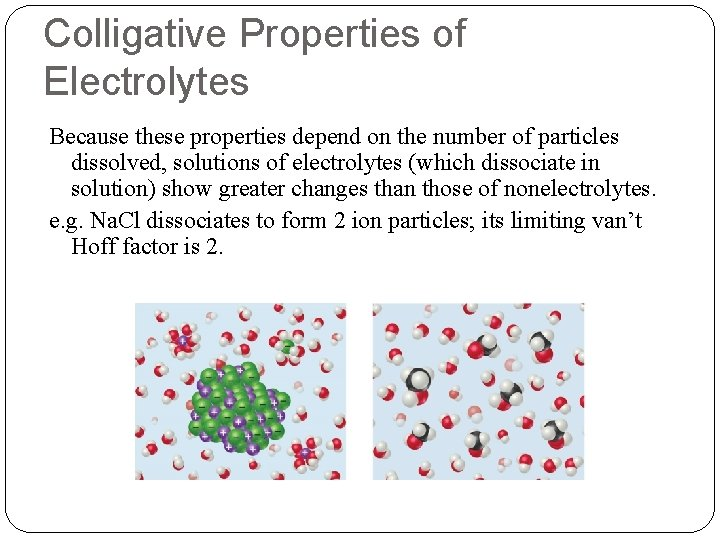Colligative Properties of Electrolytes Because these properties depend on the number of particles dissolved, solutions of electrolytes (which dissociate in solution) show greater changes than those of nonelectrolytes. e. g. Na. Cl dissociates to form 2 ion particles; its limiting van’t Hoff factor is 2.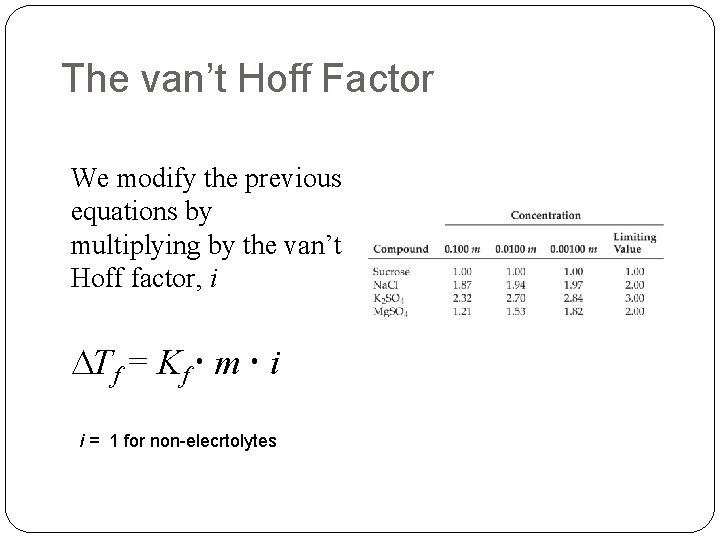The van’t Hoff Factor We modify the previous equations by multiplying by the van’t Hoff factor, i Tf = Kf m i i = 1 for non-elecrtolytes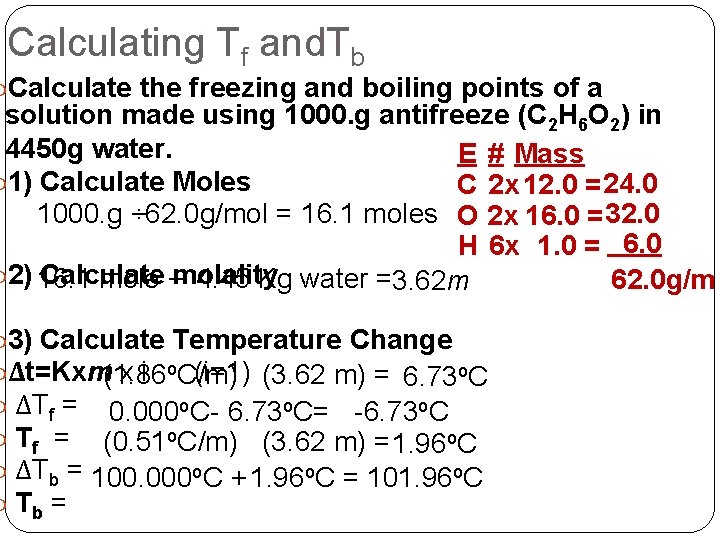Calculating Tf and. Tb Calculate the freezing and boiling points of a solution made using 1000. g antifreeze (C 2 H 6 O 2) in 4450 g water. E # Mass 1) Calculate Moles C 2 x 12. 0 = 24. 0 1000. g ÷ 62. 0 g/mol = 16. 1 moles O 2 x 16. 0 = 32. 0 H 6 x 1. 0 = 6. 0 2) 16. 1 Calculate mole ÷molality 4. 45 Kg water =3. 62 m 62. 0 g/m 3) Calculate Temperature Change Δt=Kxm(1. 86 x i o. C/m) (i=1) (3. 62 m) = 6. 73 o. C ΔTf = 0. 000 o. C- 6. 73 o. C= -6. 73 o. C Tf = (0. 51 o. C/m) (3. 62 m) = 1. 96 o. C ΔTb = 100. 000 o. C + 1. 96 o. C = 101. 96 o. C Tb =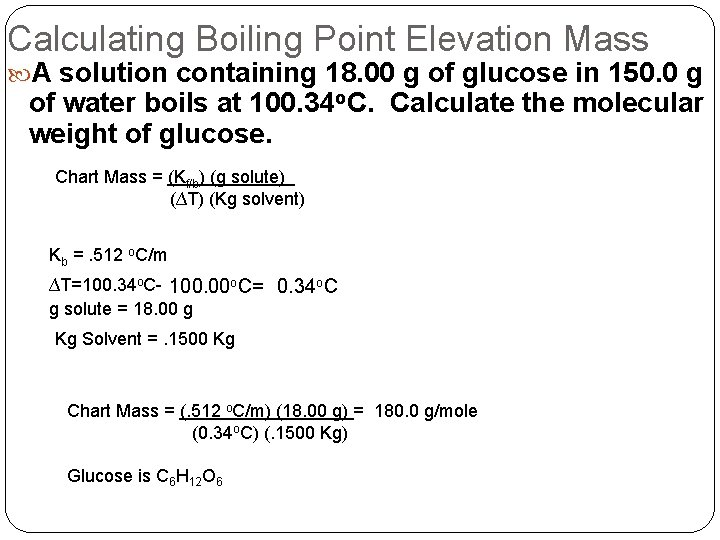Calculating Boiling Point Elevation Mass A solution containing 18. 00 g of glucose in 150. 0 g of water boils at 100. 34 o. C. Calculate the molecular weight of glucose. Chart Mass = (Kf/b) (g solute) (∆T) (Kg solvent) Kb =. 512 o. C/m ∆T=100. 34 o. C- 100. 00 o. C= 0. 34 o. C g solute = 18. 00 g Kg Solvent =. 1500 Kg Chart Mass = (. 512 o. C/m) (18. 00 g) = 180. 0 g/mole (0. 34 o. C) (. 1500 Kg) Glucose is C 6 H 12 O 6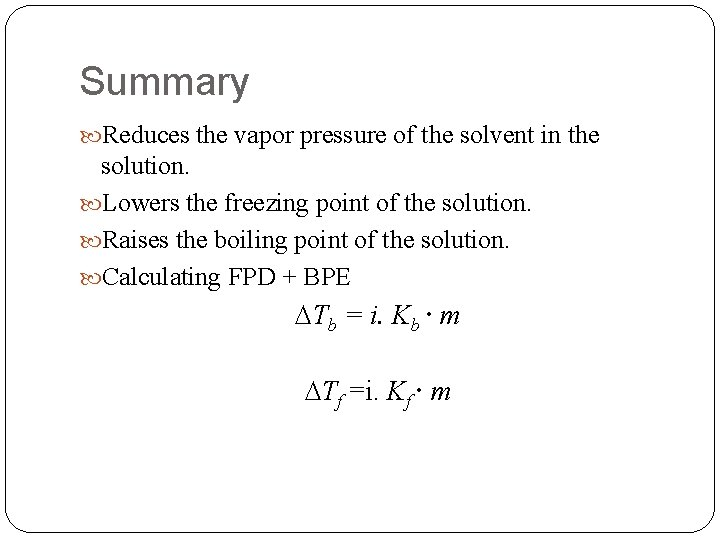Summary Reduces the vapor pressure of the solvent in the solution. Lowers the freezing point of the solution. Raises the boiling point of the solution. Calculating FPD + BPE Tb = i. Kb m Tf =i. Kf mExtra Slides The next series of slides are extra slides that we didn’t get a chance to get through. They also have more sample calculations.Ice-cream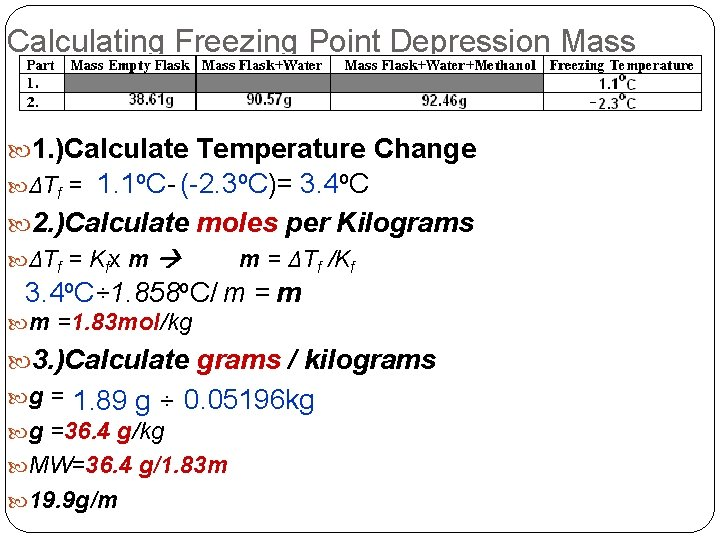Calculating Freezing Point Depression Mass 1. )Calculate Temperature Change ΔTf = 1. 1 o. C- (-2. 3 o. C)= 3. 4 o. C 2. )Calculate moles per Kilograms ΔTf = Kfx m m = ΔTf /Kf 3. 4 o. C÷ 1. 858 o. C/ m = m m =1. 83 mol/kg 3. )Calculate grams / kilograms g = 1. 89 g ÷ 0. 05196 kg g =36. 4 g/kg MW=36. 4 g/1. 83 m 19. 9 g/m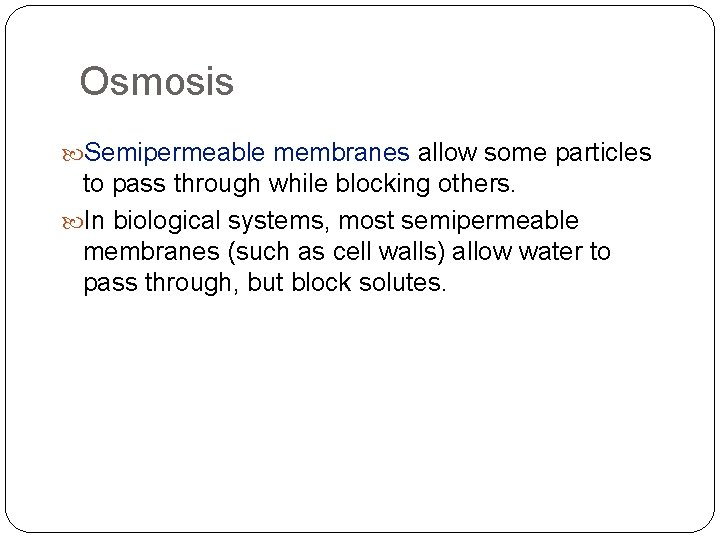Osmosis Semipermeable membranes allow some particles to pass through while blocking others. In biological systems, most semipermeable membranes (such as cell walls) allow water to pass through, but block solutes.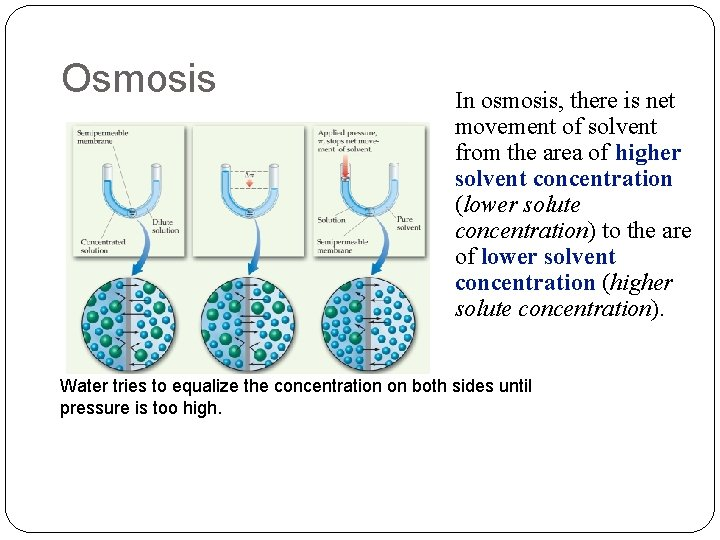Osmosis In osmosis, there is net movement of solvent from the area of higher solvent concentration (lower solute concentration) to the are of lower solvent concentration (higher solute concentration). Water tries to equalize the concentration on both sides until pressure is too high.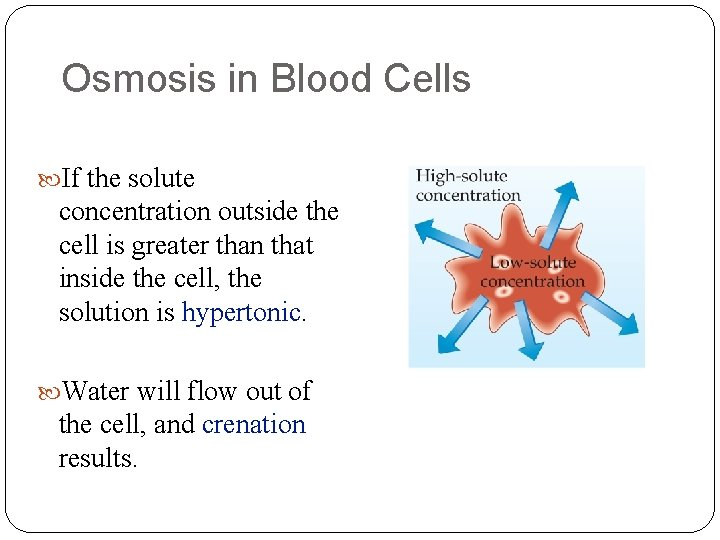Osmosis in Blood Cells If the solute concentration outside the cell is greater than that inside the cell, the solution is hypertonic. Water will flow out of the cell, and crenation results.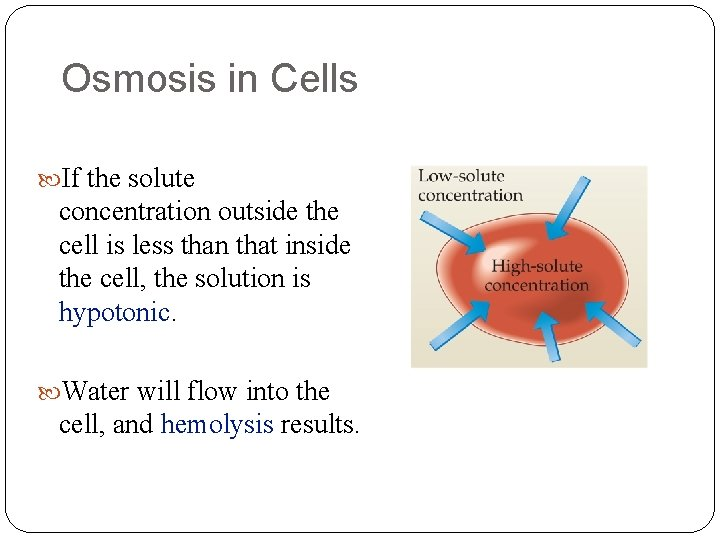Osmosis in Cells If the solute concentration outside the cell is less than that inside the cell, the solution is hypotonic. Water will flow into the cell, and hemolysis results.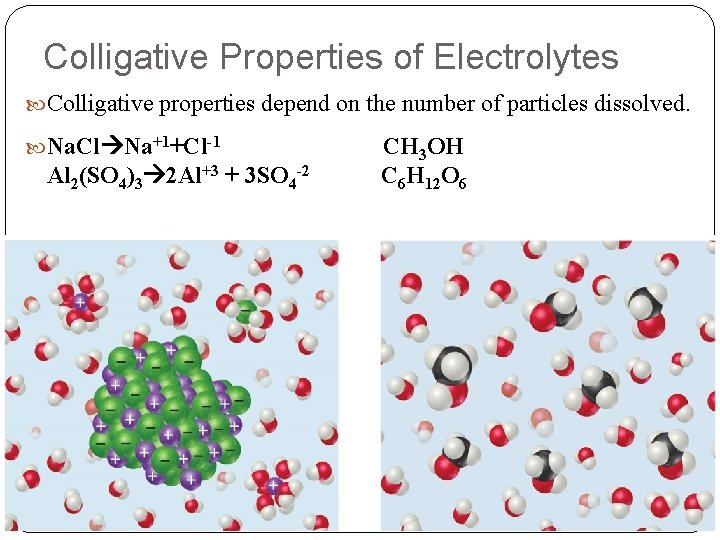Colligative Properties of Electrolytes Colligative properties depend on the number of particles dissolved. Na. Cl Na+1+Cl-1 Al 2(SO 4)3 2 Al+3 + 3 SO 4 -2 CH 3 OH C 6 H 12 O 6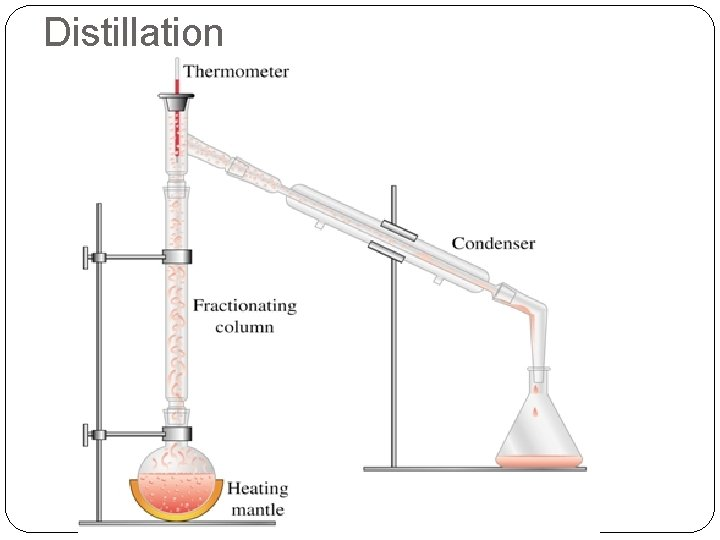DistillationDistillationOsmotic Pressure Hypertonic > 0. 92% (9. g/L) Crenation Isotonic Saline = 0. 92% (9. g/L) Hypotonic < 0. 92% (9. g/L) Rupture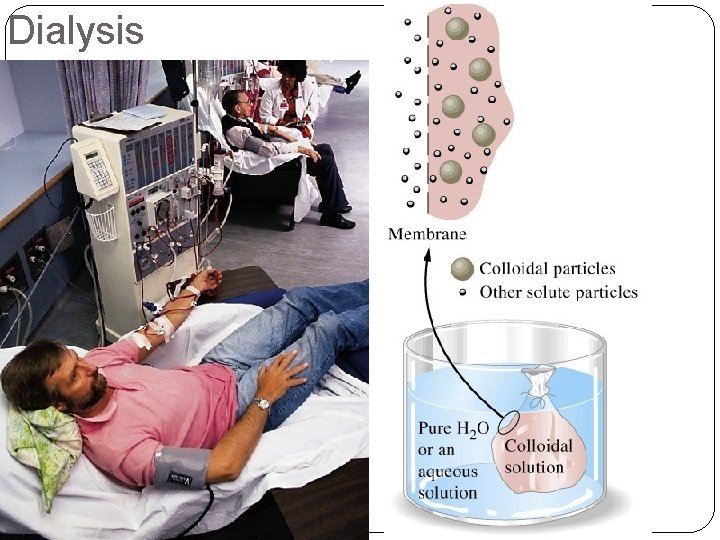Dialysis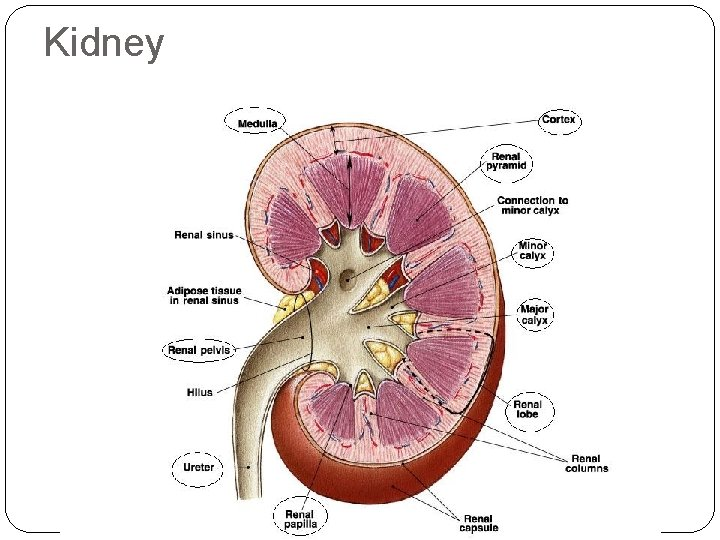Kidney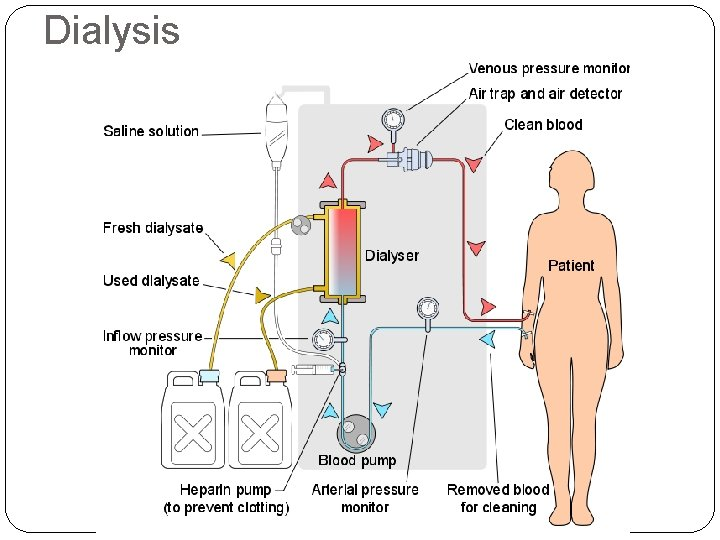Dialysis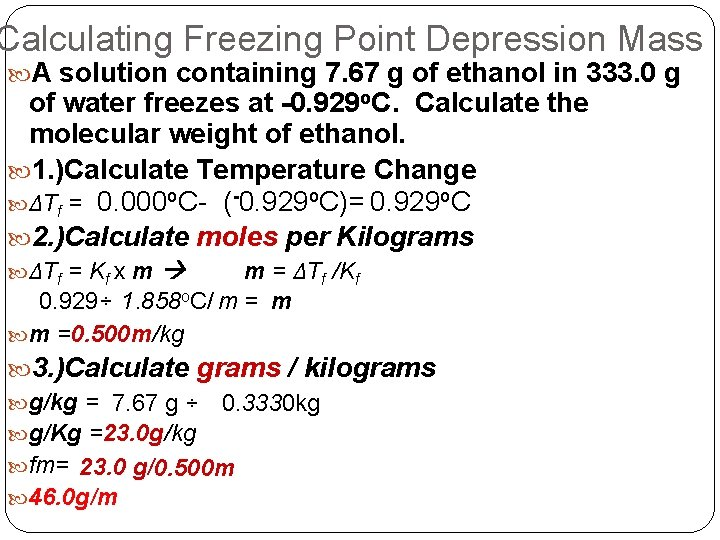Calculating Freezing Point Depression Mass A solution containing 7. 67 g of ethanol in 333. 0 g of water freezes at -0. 929 o. C. Calculate the molecular weight of ethanol. 1. )Calculate Temperature Change ΔTf = 0. 000 o. C- (-0. 929 o. C)= 0. 929 o. C 2. )Calculate moles per Kilograms ΔTf = Kf x m m = ΔTf /Kf 0. 929÷ 1. 858 o. C/ m = m m =0. 500 m/kg 3. )Calculate grams / kilograms g/kg = 7. 67 g ÷ 0. 3330 kg g/Kg =23. 0 g/kg fm= 23. 0 g/0. 500 m 46. 0 g/m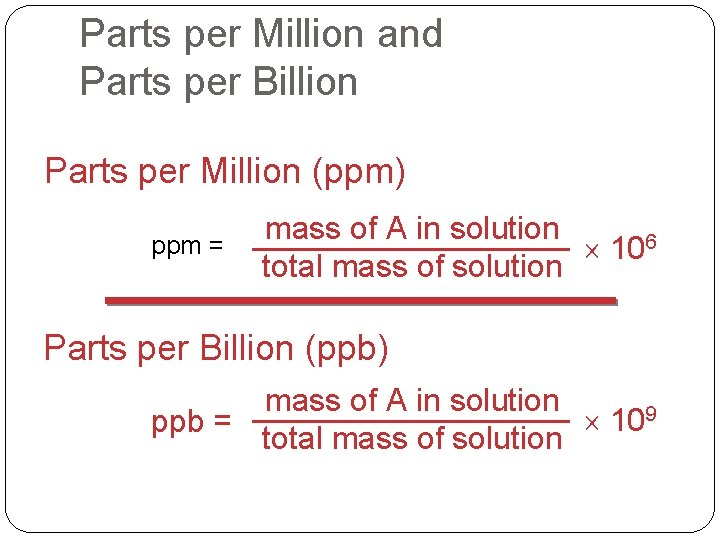Parts per Million and Parts per Billion Parts per Million (ppm) ppm = mass of A in solution 106 total mass of solution Parts per Billion (ppb) mass of A in solution 109 ppb = total mass of solution